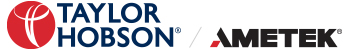Skip to content

# Surface Finish Amplitude Parameters

Monday, July 25, 2022
Tag :

## What are Surface Finish Amplitude Parameters?

Amplitude Parameters are measures of the vertical characteristics of the surface deviations.

 Surface Finish Parameters Symbols Amplitude parameters Ra, Rq, Wa, Wq, Pa, Pq Rv, Rp, Rt, Wv, Wp, Wt, Pv, Pp, Pt Rsk, Rku, Wsk, Wku, Psk, Pku Rz (JIS), Pz (JIS) Rz, Wz, Pz, Rz1max R3z, R3y (R3z1max)

Ra, Rq, Wa, Wq, Pa, Pql 1 - l 5 are consecutive and equal sampling lengths (l the sampling length corresponds to filter cut-off length λc). The evaluation length 'L' is defined as the length of profile used for assessing surface roughness parameters.

Ra: Ra is the universally recognised, and most used, international parameter of roughness. It is the arithmetic mean of the absolute departures of the roughness profile from the mean line.

Ra = 1 ∕ l ∫0| Z[x] | dx

Rq: Rq is the rms root-mean-square (rms) value of the departures of the profile from the mean line. Rq is sometimes referred to as RMS.

Wa, Wq, Pa and Pq are the corresponding parameters from the waviness and primary profiles, respectively.

Rq = √ 1 ∕ l ∫0l∣Z²[x] ∣ dx

Rv, Rp, Rt, Wv, Wp, Wt, Pv, Pp, Pt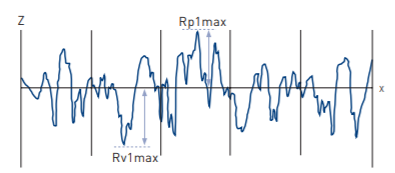Rv is the maximum depth of the profile below the mean line within the sampling length. Rp is the maximum height of the profile above the mean line within the sampling length. Rt is the maximum peak to valley height of the profile in the evaluation length. Rp1max is the largest of the individual peak to mean from each sample length. Rv1max is the largest of the individual mean to valley from each sample length. Wv, Wp, Wt, Pv, Pp and Pt are the corresponding parameters from the waviness and primary profiles, respectively.

Rsk, Rku, Wsk, Wku, Psk, Pku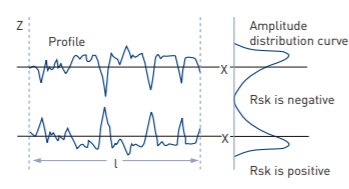Rsk – Skewness – is the measure of the symmetry of the profile about the mean line. It will distinguish between asymmetrical profiles of the same Ra or Rq.

Rsk = 1 ∕ Rq³ [ 1 ∕ l ∫l0 Z³[x] dx ]

Rku – Kurtosis – is a measure of the sharpness of the surface profile. Rsk and Rku are calculated within the sampling length.

Rku = 1 ∕ Rq4 [1 ∕ l ∫l0 Z4[x] dx ]

Wsk, Wku, Psk and Pku are the corresponding parameters from the waviness and primary profiles, respectively.

Rz (JIS), Pz (JIS)

Rz (JIS) also known as the ISO 10point height parameter in ISO 4287/1-1984, is measured on the roughness and primary profiles only and is numerically the average height difference between the five highest peaks and the five lowest valleys within the sampling length.

Rz[JIS] =  [ Zp1+Zp2+Zp3+Zp4+Zp5+Zp6 ] / 5

= 1/5 [ ∑ i=1i=5 Zp-1 - ∑i=1 i=5 Zv1 ]

Pz (JIS) is the corresponding parameter from the primary profile.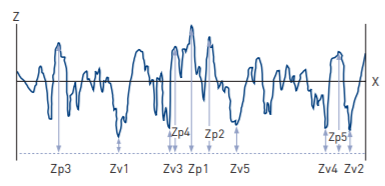Rz, Wz, Pz, Rz1max

Rz = Rp + Rv and is the maximum peak to valley height of the profile within a sampling length. Rz1max is the largest peak to valley in any sampling length within the evaluation length. Wz, Pz, are the corresponding parameters from the waviness and primary profiles respectively.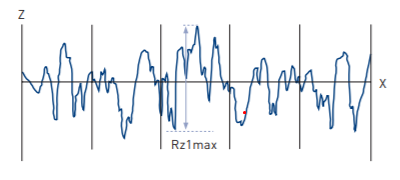R3z, R3y (R3z1max)

R3z is the vertical mean from the third highest peak to the third lowest valley in a sampling length averaged over the assessment length. DB N31007 (1983). Where N = number of sampling lengths then. R3y (R3z1max) is the largest of the R3zi, i=1...N values.

R3z = R3z1+R3z2+---+R3zN/ N

=1/N ∑ i=1i=N R3zi

Surface Finish Concepts:

How can we help?

We just need a couple of details so we can get back to you